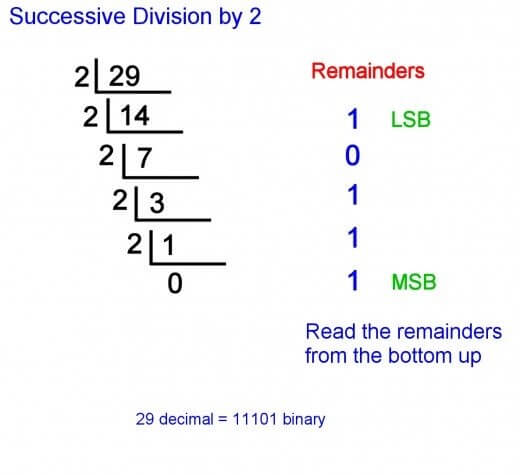# Decimal Number System

The decimal system is one of the commonly used number systems. It has a base value of 10. It is called base-10 mainly because it comprises of 10 values (0,1,2,3,4,5,6,7,8,9). Another term used is the positional number system.

## Positioning and power of each number

In any number, the left most digit is called Most Significant Bit (MSB) while the right most digit is termed as Least Significant Bit (LSB). MSB has the highest power of 10 while LSB has the lowest one. 4125 is a decimal number and it would appear as the following if broken down into powers.

4125 = 4*103 + 1*102 + 2*101 + 5*100

4125 = 4000 + 100 + 20 + 5

4125 = 4125

## LSB (Least Significant Bit) and MSB (Most Significant Bit)

In a decimal number, each digit has a significance level. The digit which has the highest level of significance is called MSB while the other with the lowest level is called LSB. If there is no digit after the decimal, the minimum bit value would be 0 and nothing will be in negative.

The number 358 will be given as

3*102 + 5*101 + 8*100

From the above example, it is obvious that the value of LSB is 0

However, the number 356.8 will have a negative LSB

356.8 = 3*102 + 5*101 + 6*100 * 8*10-1

The breakdown of 356.8 shows that the value of LSB is negative 1

## Decimal Number Conversions

Numbers in decimal form can be converted to various systems including binary, octal and hexadecimal

1. Decimal to Binary conversion

To understand the conversion better, let us consider a decimal number for conversion. Suppose that 245 has to be converted to the binary form. The binary number system has base 2 as it comprises of two values only (0 and 1).

When 245 is divided by 2, it gives the following result

Quotient of 122 and remainder of 1, repeat the process till you reach the end. We would have the following results.

Quotient of 122 and remainder of 1, divide 122 by 2

Quotient of 61 and remainder of 0, divide 61 by 2,

Quotient of 30 and remainder of 1, divide 30 by 2

Quotient of 15 and remainder of 0, divide 15 by 2,

Quotient of 7 and remainder of 1, divide 7 by 2,

Quotient of 3 and remainder of 1, divide 3 by 2

Quotient of 1 and remainder of 1.

Once you are done with the division process, compile the remainders starting from the end. Thus, the decimal value of 122 will have the following binary value.

(122)10 = (1110101)22. Decimal to Octal Conversion

The octal system has base 8 because it comprises of eight values. Here is how you need to perform the conversion process.

Suppose that the number 422 in decimal format has to be converted to octal. The following steps will be performed for conversion.

Divide 422 by 8 until the remainder is less than eight. Hence, we would get the following interpretations. Record the quotient and remainder for each step of division.

• 422 divided by 8, quotient is 52 and remainder is 6
• 52 divided by 8, quotient is 6 and remainder is 4

As the remainder is less than 8, the process will end here. Now, compile the remainders starting from the end. The number 422 in decimal form will have the following octal value.

(422)10 = (46)8

The hexadecimal number system has base 16 as it includes a total of sixteen values. Suppose that you need to convert 250 in decimal to the hexadecimal format. Here are the steps.

Divide the number by 16 until the remainder is no more divisible.

250 divided by 16, quotient is 15, remainder is 10

10 divided by 0, quotient is 0 and remainder is 10.

The process will stop when the quotient is 0. Hence, after inserting appropriate hexadecimal values, we have

(250)10 = (FA)16

## Decimal Fractions

If a number is in decimal form, it has to be converted to fractions for adding, subtracting, dividing or multiplying

Consider that we have the numbers 6.25 and 5.68 and they have to be added together. Here are the standard steps for performing this operation without using a calculator.

1. Convert both these numbers to fractions

The two numbers are 6.25 and 5.68. As both of them have two digits after the decimal, the denominator will be 100. Hence, we would have the following fractions.

625/100 and 568/100.

Both the numbers have 100 as the denominator so no LCM is needed.

1. Perform the mathematical operation

When we add the two fractions written above, the following result will be obtained

625/100 + 568/100

= 1193/100

=11.93

Apart from addition, the other mathematical operations can also be performed in a similar manner. For instance, the same numbers will be multiplied by performing the following steps.

6.25*5.68

Convert both the numbers to decimal fractions.

625/100 * 568/100

355000 / 10000

= 35.5

Reference

#### Need Custom Calculator?

With Over Online Tools, eCalculator.co Helping Millions of Students, Teachers, Businessmen & Nutritionists Every Month.